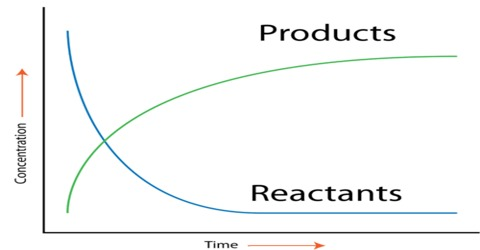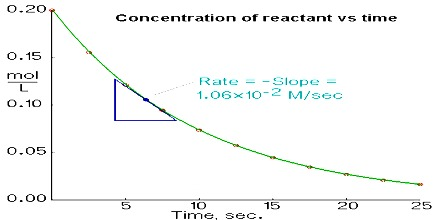Chemistry

# Definition of Rate of a ReactionDefinition of Rate of a Reaction

The rate of a reaction (rate) is the amount of reactant used up or the amount of product formed in unit time. In application in chemistry the amount is expressed in moles, or more usually in mol L-1. The rate of a chemical reaction is the decrease of concentration (in mol L-1) of the reactant or increase in the concentration of the product in unit time. The unit of the rate is usually expressed as mol L-1 s-1 (or min-1, h-1 etc. depending on the ‘fastness’ or ‘slowness’ of the reaction). Let us consider the hypothetical reaction

A → BAs the reaction proceeds the concentration of A decreases and that of B increases. If the increase and decrease are simply stated as the change in concentration then,

Rate = – ∆[A] / ∆t … … … (1)

= + ∆[B] / ∆t … … … (2)

Note the different signs in the two expressions. In the equation (l) the negative sign indicates that the concentration of the reactant A decreases with the progress of reaction, and the positive sign in equation (2) signifies that the concentration of the product B increases with time. Reaction rate is  the speed at which a chemical reaction proceeds. It is often expressed in terms of either the concentration (amount per unit volume) of a product that is formed in a unit of time or the concentration of a reactant that is consumed in a unit of time.